• 二进制浮点数规格化
2022-01-09 12:37:07

##1.二进制浮点数
先分别将整数和小数部分化为二进制，然后整数部分 + 小数点 + 小数部分 + 补的0即可

例：将12.25化为二进制
12的二进制形式为1100，0.25的二进制为0.01，所以结果为1100.01。
##2.IEEE754存储格式

1. 浮点数化为二进制后，将其小数点移到第一位1的后面，并把第一位1和小数点去掉，余下部分为该格式的尾数（去掉1是因为规格化规范为1.XXX，可把1去掉增加一位精度）。
2. 计算127(此为32位的偏移量，64位的偏移量为1023) + 之前小数点移动的位数，再将其化为二进制，此为该格式的阶码
3. 判断该数的正负，正为0负为1，此为该格式的符号位
4. 把符号位，阶码，尾数连起来，即为所求的IEEE754格式。
5. 存储格式为：1位符号位 + 8位阶码 + 23位尾数（32位）或1位符号位 + 11位阶码 + 52位尾数（64位）

例：将12.25化为IEEE754规范下的二进制格式

1. 1100.01化为1.10001，小数点移动了3位，可得尾数为10001000000000000000000
2. 计算得阶码为130，化为二进制为10000010
3. 该数为正数，符号位为0，则最终IEEE754格式为0 10000010 10001000000000000000000算法
更多相关内容
• 二进制浮点数以及二进制浮点数算术运算二进制浮点数表示半精度浮点数单精度浮点数双精度浮点数特殊情况浮点数的运算步骤一、对阶二、尾数运算三、结果规格化左规操作右规操作四、 舍入处理五、 溢出判断六、例子...

# 二进制浮点数表示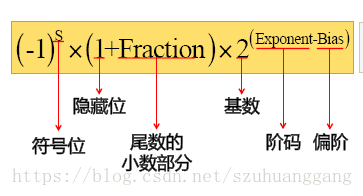阶码（指数）就是指数位存储的值，而偏阶（移码）则不同精度的浮点数的偏阶也各不相同，具体可以查看指数偏差
B i a s = 2 k − 1 − 1 Bias=2^{k-1}-1 , 其中k是指数中的位数。

## 半精度浮点数

半精度浮点数 是一种被计算机使用的二进制浮点数据类型。半精度浮点数使用2个字节（16位）来存储。
在IEEE 754-2008中，它被称作binary16。这种数据类型只适合存储对精度要求不高的数字，不适合用来计算。

IEEE 754 标准指定了一个 binary16 要有如下的格式：
Sign bit（符号位）： 1 bit
Exponent width（指数位宽）： 5 bits
Significand precision（尾数精度）： 11 bits （有10位被显式存储）

按如下顺序排列：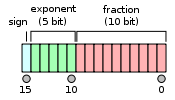除非指数位全是0，否则就会假定隐藏的起始位是1。因此只有10位尾数在内存中被显示出来，而总精度是11位。据IEEE 754的说法，虽然尾数只有10位，但是尾数精度是11位的(log10(211) ≈ 3.311 十进制数).

## 单精度浮点数

单精度浮点数 格式是一种数据类型，在计算机存储器中占用4个字节（32 bits），利用“浮点”（浮动小数点）的方法，可以表示一个范围很大的数值。

第1位表示正负，中间8位表示指数，后23位储存有效数位（有效数位是24位）。

第一位的正负号0代表正，1代表负。

中间八位共可表示 2 8 = 256 2^8=256 个数，指数可以是二补码；或0到255，0到126代表-127到-1，127代表零，128-255代表1-128。

有效数位最左手边的1并不会储存，因为它一定存在（二进制的第一个有效数字必定是1）。换言之，有效数位是24位，实际储存23位。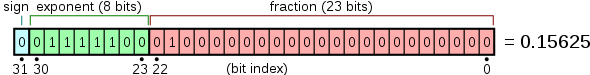s i g n = + 1 sign = +1
e x p o n e n t = ( − 127 ) + 124 = − 3 exponent = (-127)+124=-3
f r a c t i o n = 1 + 2 − 2 = 1.25 fraction=1+2^{-2}=1.25
v a l u e = ( + 1 ) × 1.25 × 2 − 3 = + 0.15625 value=(+1)\times1.25\times2^{-3}=+0.15625

## 双精度浮点数

双精度浮点数（double）是计算机使用的一种资料类型。比起单精度浮点数，双精度浮点数(double)使用 64 位（8字节） 来存储一个浮点数。 它可以表示二进位制的53位有效数字，其可以表示的数字的绝对值范围为 [ 2 − 1024 , 2 1024 ] [2^{-1024}, 2^{1024}]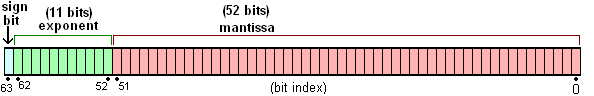## 特殊情况

以双精度浮点数为例，说明一些特殊情况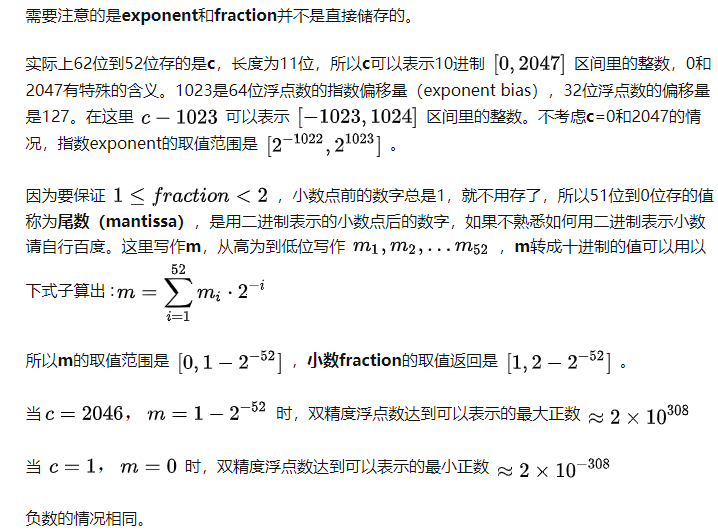当指数exponent全为0或者全为1时，有特殊含义，有以下四种情况，
1、 e x p o n e n t = 0 , f r a c t i o n = 0 ⇒ ± 0 exponent=0, fraction=0 \Rightarrow \pm0
2、 e x p o n e n t = 0 , f r a c t i o n ≠ 0 ⇒ 非 正 规 形 式 的 浮 点 数 exponent=0, fraction\neq0 \Rightarrow 非正规形式的浮点数
3、 e x p o n e n t = 2047 , f r a c t i o n = 0 ⇒ ± ∞ exponent=2047, fraction=0 \Rightarrow \pm\infty
4、 e x p o n e n t = 2047 , f r a c t i o n ≠ 0 ⇒ N a N exponent=2047, fraction\neq0 \Rightarrow NaN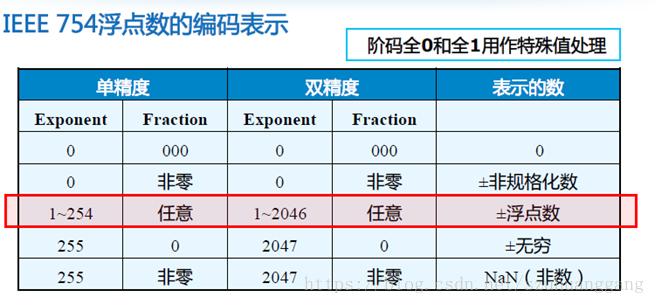# 浮点数的运算步骤

浮点数的加减运算一般由以下五个步骤完成：对阶、尾数运算、规格化、舍入处理、溢出判断

## 一、对阶

所谓对阶是指将两个进行运算的浮点数的阶码对齐的操作。对阶的目的是为使两个浮点数的尾数能够进行加减运算。因为，当进行 M x ⋅ 2 E x M_x·2^{E_x} M y ⋅ 2 E y M_y·2^{E_y} 加减运算时，只有使两浮点数的指数值部分相同，才能将相同的指数值作为公因数提出来，然后进行尾数的加减运算。对阶的具体方法是：首先求出两浮点数阶码的差，即 Δ E = E x − E y \Delta E = E_x - E_y ，将小阶码加上 Δ E \Delta E ，使之与大阶码相等，同时将小阶码对应的浮点数的尾数右移相应位数，以保证该浮点数的值不变。几点注意：

（1）对阶的原则是小阶对大阶，之所以这样做是因为若大阶对小阶，则尾数的数值部分的高位需移出，而小阶对大阶移出的是尾数的数值部分的低位，这样损失的精度更小。

（2）若 Δ E \Delta E ＝0，说明两浮点数的阶码已经相同，无需再做对阶操作了。

（3）采用补码表示的尾数右移时，符号位保持不变。

（4）由于尾数右移时是将最低位移出，会损失一定的精度，为减少误差，可先保留若干移出的位，供以后舍入处理用。

## 二、尾数运算

尾数运算就是进行完成对阶后的尾数相加减。这里采用的就是我们前面讲过的纯小数的定点数加减运算。

## 三、结果规格化

在机器中，为保证浮点数表示的唯一性，浮点数在机器中都是以规格化形式存储的。对于IEEE754标准的浮点数来说，就是尾数必须是1.M的形式。由于在进行上述两个定点小数的尾数相加减运算后，尾数有可能是非规格化形式，为此必须进行规格化操作。

规格化操作包括左规和右规两种情况。

### 左规操作

将尾数左移，同时阶码减值，直至尾数成为 1. M 1.M 的形式。例如，浮点数 0.0011 ∗ 2 5 0.0011*2^5 是非规格化的形式，需进行左规操作，将其尾数左移3位，同时阶码减3，就变成 1.1100 ∗ 2 2 1.1100*2^2 规格化形式了。

### 右规操作

将尾数右移1位，同时阶码增1，便成为规格化的形式了。要注意的是，右规操作只需将尾数右移一位即可，这种情况出现在尾数的最高位（小数点前一位）运算时出现了进位，使尾数成为 10. x x x x 10.xxxx 11. x x x x 11.xxxx 的形式。例如， 10.0011 ∗ 2 5 10.0011*2^5 右规一位后便成为 1.00011 ∗ 2 6 1.00011*2^6 的规格化形式了。

## 四、 舍入处理

浮点运算在对阶或右规时，尾数需要右移，被右移出去的位会被丢掉，从而造成运算结果精度的损失。为了减少这种精度损失，可以将一定位数的移出位先保留起来，称为保护位，在规格化后用于舍入处理。

IEEE754标准列出了四种可选的舍入处理方法：

（1）就近舍入（round to nearest）这是标准列出的默认舍入方式，其含义相当于我们日常所说的“四舍五入”。例如，对于32位单精度浮点数来说，若超出可保存的23位的多余位大于等于 100 … 01 100…01 ，则多余位的值超过了最低可表示位值的一半，这种情况下，舍入的方法是在尾数的最低有效位上加1；若多余位小于等于 011 … 11 011…11 ，则直接舍去；若多余位为 100 … 00 100…00 ，此时再判断尾数的最低有效位的值，若为0则直接舍去，若为1则再加1。

（2） + ∞ +∞ 舍入（round toward + ∞ +∞ ）对正数来说，只要多余位不为全0，则向尾数最低有效位进1；对负数来说，则是简单地舍去。

（3） − ∞ -∞ 舍入（round toward − ∞ -∞ ）与朝 + ∞ +∞ 舍入方法正好相反，对正数来说，只是简单地舍去；对负数来说，只要多余位不为全0，则向尾数最低有效位进1。

（4）朝0舍入（round toward 0）

即简单地截断舍去，而不管多余位是什么值。这种方法实现简单，但容易形成累积误差，且舍入处理后的值总是向下偏差。

## 五、 溢出判断

与定点数运算不同的是，浮点数的溢出是以其运算结果的阶码的值是否产生溢出来判断的。若阶码的值超过了阶码所能表示的最大正数，则为上溢，进一步，若此时浮点数为正数，则为正上溢，记为 + ∞ +∞ ，若浮点数为负数，则为负上溢，记为 − ∞ -∞ ；若阶码的值超过了阶码所能表示的最小负数，则为下溢，进一步，若此时浮点数为正数，则为正下溢，若浮点数为负数，则为负下溢。正下溢和负下溢都作为0处理。

要注意的是，浮点数的表示范围和补码表示的定点数的表示范围是有所不同的，定点数的表示范围是连续的，而浮点数的表示范围可能是不连续的。

## 六、例子

f l o a t      a = 0.3 ; b = 1.6 float \ \ \ \ a=0.3;b=1.6 ;

a = ( 0.3 ) 10 = ( 0011   1110   1001   1001   1001   1001   1001   1010 ) 2 a=(0.3)_{10}=(0011\ 1110\ 1001\ 1001\ 1001\ 1001\ 1001\ 1010)_2
S a = 0      E a = 011   1110   1      M a = 1.001   1001   1001   1001   1001   1010 S_a=0\ \ \ \ E_a=011\ 1110\ 1\ \ \ \ M_a=1.001\ 1001\ 1001\ 1001\ 1001\ 1010

b = ( 1.6 ) 10 = ( 0011   1111   1100   1100   1100   1100   1100   1101 ) 2 b=(1.6)_{10}=(0011\ 1111\ 1100\ 1100\ 1100\ 1100\ 1100\ 1101)_2
S b = 0      E b = 011   1111   1     M b = 1.100   1100   1100   1100   1100   1101 S_b=0\ \ \ \ E_b=011\ 1111\ 1\ \ \ M_b=1.100\ 1100\ 1100\ 1100\ 1100\ 1101

a + b = ? a+b=?

### 二进制浮点数加法

第一步：对阶

∵ E a < E b     E b − E a = 2 ∵ E_a < E_b\ \ \ E_b-E_a=2

∴ M a 要 调 整 为 0.0   1001   1001   1001   1001   1001   10         10 ∴ Ma要调整为 0.0\ 1001\ 1001\ 1001\ 1001\ 1001\ 10\ \ \ \ \ \ \ 10

E = 011    1111    1 E=011\ \ 1111\ \ 1

第二步：尾数运算

0.01001100110011001100110 \ \ \ \ 0.01001100110011001100110
+   1.10011001100110011001101 +\ 1.10011001100110011001101
= 1.11100110011001100110011 =1.11100110011001100110011

第三步：规格化

1.11100110011001100110011 1.11100110011001100110011 ‬已经是个规格化数据了

第四步：舍入处理

由于在对阶时， M a M_a 有右移，且第一次最高为1，第二次为0，所以按"0舍1入"，尾数运算结果调整为 1.11100110011001100110100 1.11100110011001100110100

第五步：溢出判断

没有溢出，阶码不调整，所以最后的结果为

a + b = ( 0    01111111    11100110011001100110100 ) 2 = ( 0011   1111   1111   0011   0011   0011   0011   0100 ) 2 = ( 3 F F 33334 ) 16 a+b=(0\ \ 01111111\ \ 11100110011001100110100)_2=(0011\ 1111\ 1111\ 0011\ 0011\ 0011\ 0011\ 0100)_2=(3FF33334)_{16}

转为10进制

a + b = 1.90000010 a+b=1.90000010

### 二进制浮点数减法

b − a = ? b-a=?
第一步：对阶

跟上面加法一样

第二步：尾数运算

1.10011001100110011001101 \ \ \ \ 1.10011001100110011001101

−   0.01001100110011001100110 -\ 0.01001100110011001100110

= 1.01001100110011001100111 =1.01001100110011001100111

第三步：规格化

1.01001100110011001100111 1.01001100110011001100111 已经是个规格化数据了

第四步：舍入处理

由于在对阶时， M a M_a 有右移，且第一次最高为1，第二次为0，所以按"0舍1入"，尾数运算结果调整为 1.01001100110011001100110 1.01001100110011001100110

第五步：溢出判断

没有溢出，阶码不调整，所以最后的结果为

a − b = ( 0    01111111    01001100110011001100110 ) 2 = ( 0011    1111    1010    0110    0110    0110    0110    0110 ) 2 = ( 3 F A 66666 ) 16 a-b=(0\ \ 01111111\ \ 01001100110011001100110)2=(0011\ \ 1111\ \ 1010\ \ 0110\ \ 0110\ \ 0110\ \ 0110\ \ 0110)_2=(3FA66666)_{16}

转为10进制

a − b = 1.29999995 a-b=1.29999995

### 二进制浮点数乘法

浮点数的乘法分为以下几个步骤：

计算符号位：通过异或操作计算符号位，若两个操作数符号位相同，则结果符号位为0，否则结果符号为1
计算原始尾数：两个操作数的尾数相乘（注意，这里是1.M * 1.M），得到原始尾数
计算原始指数：将两个操作数的指数（这里指的是指数幂次方，也就是阶码-移码后得到的数）相加，得到原始指数
规格化与舍入：对原始尾数和原始指数进行规格化，获得结果的指数，再对尾数进行舍入，获得结果的尾数

f l o a t      X = − 0.3 ; Y = 500.25 float \ \ \ \ X=-0.3;Y=500.25 ;

X = ( − 0.3 ) 10 = ( 0.010011001100110011... 循 环 ) 2 = ( 1.00110011... 循 环 ) 2 ∗ 2 − 2 = ( 1    01111101    00110011001100110011010 ) 2 ( 这 里 对 无 限 循 环 小 数 有 舍 入 处 理 ) X=(-0.3)_{10}=(0.010011001100110011...循环)_{2}=(1.00110011...循环)_2 * 2^{-2}=(1\ \ 01111101\ \ 00110011001100110011010)_2(这里对无限循环小数有舍入处理)
Y = ( 500.25 ) 10 = ( 111110100.01 ) 2 = ( 1.1111010001 ) 2 ∗ 2 8 = ( 0    10000111    11110100010000000000000 ) 2 Y=(500.25)_{10}=(111110100.01)_2=(1.1111010001)_2*2^{8}=(0\ \ 10000111\ \ 11110100010000000000000)_2

X ∗ Y = ( X s ∗ Y s ) ∗ 2 X E + Y E X*Y=(X_s*Y_s)*2^{X_E+Y_E}
X E = − 2             X s = 1.00110011001100110011010 X_E=-2\ \ \ \ \ \ \ \ \ \ \ X_s=1.00110011001100110011010
Y E = 8                  Y s = 1.1111010001 Y_E=8\ \ \ \ \ \ \ \ \ \ \ \ \ \ \ \ Y_s=1.1111010001
X s          1.0011001100110011001101 \ \ X_s\ \ \ \ \ \ \ \ 1.0011001100110011001101
∗   Y s      ∗                          1.1111010001 *\ Y_s\ \ \ \ *\ \ \ \ \ \ \ \ \ \ \ \ \ \ \ \ \ \ \ \ \ \ \ \ 1.1111010001
= 10.01011000010011001100111001011101 =10.01011000010011001100111001011101

X ∗ Y = 10.01011000010011001100111001011101 ∗ 2 6 X*Y=10.01011000010011001100111001011101*2^6
= 1.001011000010011001100111001011101 ∗ 2 7 ( 右 规 操 作 ) \ \ \ \ \ \ \ \ \ \ \ \ =1.001011000010011001100111001011101*2^7(右规操作)

结果二进制就为(注意，上面的指数7要加上移码才是存在指数位中的阶码，即7+127=134)
( 1    10000110    00101100001001100110100 ) 2 (1\ \ 10000110\ \ 00101100001001100110100)_2
至于最后的尾数是怎么得到，是由原始尾数（X和Y的尾数相乘后得到）经过舍入得到

### 二进制浮点数除法

除法与乘法相差不大，变更为指数相减，尾数相除。

f l o a t      X = 91.34375 ; Y = 0.14453125 float \ \ \ \ X=91.34375;Y=0.14453125 ;

X = ( 91.34375 ) 10 = ( 1011011.01011 ) 2 = ( 1.01101101011 ) 2 ∗ 2 6 = ( 0    10000101    01101101011000000000000 ) 2 X=(91.34375)_{10}=(1011011.01011)_{2}=(1.01101101011)_2 * 2^{6}=(0\ \ 10000101\ \ 01101101011000000000000)_2
Y = ( 0.14453125 ) 10 = ( 0.00100101 ) 2 = ( 1.00101 ) 2 ∗ 2 8 = ( 0    01111100    00101000000000000000000 ) 2 Y=(0.14453125)_{10}=(0.00100101)_2=(1.00101)_2*2^{8}=(0\ \ 01111100\ \ 00101000000000000000000)_2

X / Y = ( X s / Y s ) ∗ 2 X E − Y E = ( X s / Y s ) ∗ 2 6 − ( − 3 ) X/Y=(X_s/Y_s)*2^{X_E-Y_E}=(X_s/Y_s)*2^{6-(-3)}
X E = 6                   X s = 1.01101101011 X_E=6\ \ \ \ \ \ \ \ \ \ \ \ \ \ \ \ \ X_s=1.01101101011
Y E = − 3                  Y s = 1.00101 Y_E=-3\ \ \ \ \ \ \ \ \ \ \ \ \ \ \ \ Y_s=1.00101
X s          1.01101101011 \ \ X_s\ \ \ \ \ \ \ \ 1.01101101011
/   Y s      /    1.00101 /\ Y_s\ \ \ \ /\ \ 1.00101
= 1.001111 =1.001111

9 + 127 = 136 9+127 = 136

( 0    10001000    00111100000000000000000 ) 2 (0\ \ 10001000\ \ 00111100000000000000000)_2

# 浮点运算逻辑电路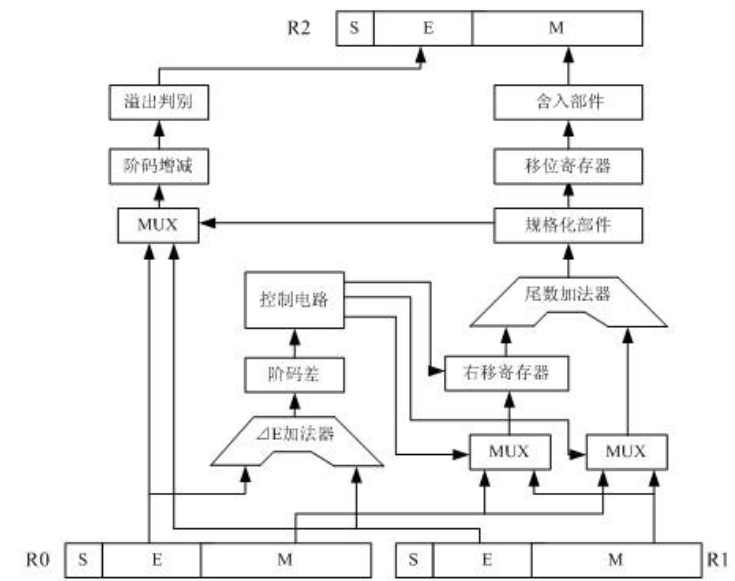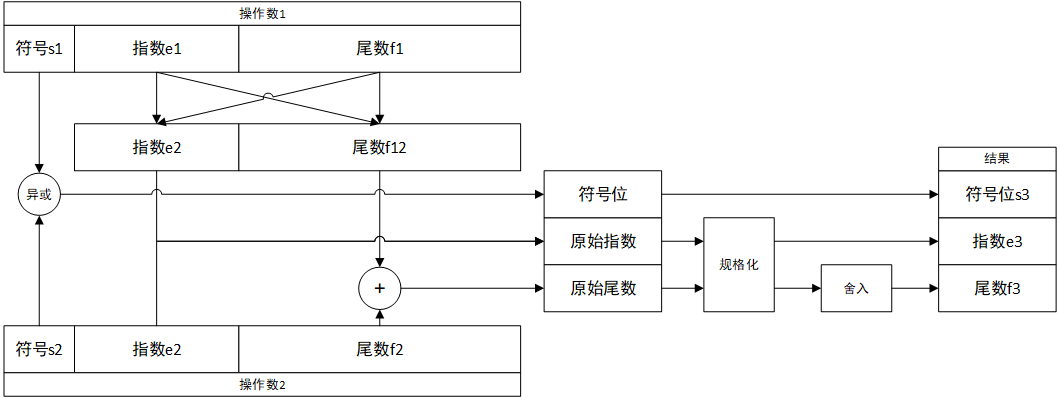# Reference

https://zhuanlan.zhihu.com/p/58731780
https://www.yuejianzun.xyz/2019/05/28/%E6%B5%AE%E7%82%B9%E6%95%B0%E5%A4%84%E7%90%86/
https://www.cnblogs.com/yilang/

展开全文• ## 二进制浮点数的加减法运算

千次阅读 多人点赞 2019-09-27 11:45:55
二进制浮点数规格化表示形式定义为N=2E⋅MN=2^E·MN=2E⋅M其中MMM称为尾数，EEE称为阶码 例如二进制浮点数11.011011的规格化表示形式为：2+0010×0.110110112^{+0010}×0.110110112+0010×0.11011011该浮点数在...

二进制浮点数的规格化表示形式定义为 N = 2 E ⋅ M N=2^E·M 其中 M M 称为尾数， E E 称为阶码

例如二进制浮点数11.011011的规格化表示形式为： 2 + 0010 × 0.11011011 2^{+0010}×0.11011011 该浮点数在计算机中存储为：

00100011011011

各个二进制位代表的含义为：
阶符E | 阶码E | 尾符M | 尾码M
00      010    00      11011011


现假设有两浮点数 X X Y Y
X : 2 + 010 × 0.11011011 X:2^{+010}×0.11011011 Y ： 2 + 100 × − 0.10101100 Y：2^{+100}×-0.10101100
X X Y Y 在计算机中表示为：

    阶符E | 阶码E | 尾符M | 尾码M
X   00      010    00      11011011
Y   00      100    11      01010100


要在计算机中实现加减法运算要执行5个步骤：

### 1. 对阶

对阶的目的是为了使两个阶数不同的浮点数变换到为可以直接相加，例如在十进制中 1.2 × 1 0 2 1.2×10^2 2.3 × 1 0 3 2.3×10^3 的尾数是不能直接相加的，必须转换成相同的阶数才可以相加
1.2 × 1 0 2 +   2.3 × 1 0 3 ‾ 3.3 × 1 0 ? ⟹ 0.12 × 1 0 3 +   2.3    × 1 0 3 ‾ 2.42 × 1 0 3 \begin{aligned} & \quad 1.2 × 10^2 \\ & \underline{+\ 2.3 × 10^3}\\ & \quad 3.3 × 10^?\\ \end{aligned}\Longrightarrow \begin{aligned} & \quad 0.12 × 10^3 \\ & \underline{+\ 2.3 \ \ × 10^3}\\ & \quad 2.42 × 10^3\\ \end{aligned}

#### 1.1 取大阶

保留两个浮点数中阶码较大的那一个浮点数的阶数，较小阶码的浮点数的阶数在之后需要对齐大阶，取大阶的公式为： E m a x = max ⁡ ( E X , E Y ) E_{max} = \max(E_X,E_Y) 之所以是取大阶而不是取小阶是因为，浮点数往小阶对其后，小数点会进入尾数之中，与上面举的例子不同，这种浮点数形式是无法在计算机中存储的。

取大阶举例，对 X X Y Y 取大阶：
E m a x = max ⁡ ( E X , E Y ) = E Y E_{max} = \max(E_X,E_Y)=E_Y

#### 1.2 求阶差

求出阶差后可以根据阶差对阶码小的浮点数的尾数进行调整，求阶差公式： Δ E = ∣ E X − E Y ∣ 补 \Delta E = |E_{X}-E_{Y}|_{补}

求阶差举例，对 X X Y Y 求阶差：
Δ E = ∣ E X − E Y ∣ 补 = ∣ E X 补 − E Y 补 ∣ = ∣ E X 补 + ( − E Y ) 补 ∣ = ∣ 00010 + 11100 ∣ = ∣ 11110 ∣ = 2 \begin{aligned} \Delta E=& |E_{X}-E_{Y}|_{补}\\ =& |E_{X补}-E_{Y补}|\\ =& |E_{X补}+(-E_{Y})_{补}|\\ =& |00010 + 11100|\\ =& |11110|=2 \end{aligned}

#### 1.3 对阶

1.2求阶差 Δ E ≠ 0 \Delta E \neq 0 ，则执行对阶操作，将阶码值较小的浮点数尾数右移 Δ E \Delta E 位，使得两个浮点数的阶码值相等。

由于进行了右移，对阶操作往往会损失阶码值较小的浮点数的一部分精度从而产生计算误差，若要减小计算误差，则要将右移过程中损失的尾数值保留下了以供后面3.2.2左规格化和4.2舍入步骤使用，这里不细说。

对阶举例，对 X X Y Y 对阶：
已知 X X Y Y 的大阶阶码 E m a x = E Y E_{max}=E_Y ，阶差 Δ E = 2 \Delta E=2 。即 E X E_X 位向 E Y E_Y 对齐，且 M X M_X 右移 Δ E \Delta E 位：
00010 ‾ 00 11011011 ‾ ⟹ 00100 ‾ 00 00110110 ‾ \underline{00010}00\underline{11011011}\Longrightarrow\underline{00100}00\underline{00110110} 对阶后的 X X Y Y :

    阶符E | 阶码E | 尾符M | 尾码M
X   00      100    00      00110110
Y   00      100    11      01010100


对阶前的 X X Y Y :

    阶符E | 阶码E | 尾符M | 尾码M
X   00      010    00      11011011
Y   00      100    11      01010100


### 2. 尾数运算

#### 2.1 尾数运算

对阶操作完成之后浮点数的尾数就可以进行运算了，公式可以表示为： M = [ ( M X ) ± ( M Y ) ] 补 M=[(M_X)\pm(M_Y)]_补

尾数运算举例，对 X X Y Y 的尾数进行运算：
M = [ ( M X ) + ( M Y ) ] 补 = ( M X ) 补 + ( M Y ) 补 M=[(M_X)+(M_Y)]_补=(M_X)_补+(M_Y)_补
00100 0000110110 ‾ +   00100 1101010100 ‾ ‾ 00100 1110001010 ‾ \begin{aligned} & \quad 00100\underline{0000110110} \\ & \underline{+\ 00100\underline{1101010100}}\\ & \quad 00100\underline{1110001010}\\ \end{aligned}

### 3. 规格化

#### 3.1 判断尾数溢出

双符号位的判断溢出方法：二进制数的符号一定为00(正)或11(负)，运算后若符号位变为01则是正溢出，变为10则是负溢出，二进制数相加结果符号位溢出判断如下表所示：

正数负数正溢负溢
00110110

可以简记为，符号位异或的结果为0则溢出，为1则不溢出。

#### 3.2 左右规格化

##### 3.2.1 右规格化

若尾数加减后的结果 M M 溢出则执行右规格化操作，即尾数右移一位且阶码加1，表示为 M ≫ 1 , E + 1 M\gg 1,E+1

##### 3.2.2 左规格化

若尾数加减后的结果 M M 不溢出则执行左规格化操作，即尾数左移K位且阶码减K，表示为 M ≪ K , E − K M\ll K,E-K 其中 K K 表示将尾数数值最高位变为与尾数符号位不同值需要执行左移的次数。注意此处的左移要包括1.3对阶时被移出低位的尾数值。

左规格化的目的是为了提高尾数的精度，例如， M = 001001110001010 M=001001110001010 转为真值表示为 2 4 × − 0.01110110 2^4×-0.01110110 ，由于在计算机中尾数的存储位数有限，这种表示方法就会造成精度的下降，改为 2 3 × − 0.11101100 2^3×-0.11101100 就可以多存一个位的数据

尾数运算举例，对 M M 的尾数进行左规格化：
尾数左移1位(括号中为对阶时被移出低位的尾数值)： 1 11 ‾ 0001010 ( 11 ) ⟹ 1 10 ‾ 0010101 ( 1 ) 1\underline{11}0001010(11)\Longrightarrow 1\underline{10}0010101(1) 阶码减1： 00100 − 00001 = 00011 00100-00001=00011

### 4. 舍入

#### 4.1 判断阶码溢出

如果在上一步执行的是右规格化，则要判断阶码是否上溢，若执行的是左规格化，则要判断阶码是否下溢。

判断方法与3.1一致，符号位异或的结果为0则溢出，为1则不溢出。右规格化若上溢则置上溢标志并且报运算结果溢出错误，左规格化若下溢则置机器零到5.4输出结果

#### 4.2 舍入

若左右规格化的步骤执行后结果不溢出，则进行舍入处理。

在执行3.2.1右规格化和1.3对阶时，尾数低位上的数值会被移除掉使得浮点数精度下降。舍入就是用来弥补被移除掉的精度，当移除掉的低位数值最高位为1时，在规格化后的尾数末尾加1，若加1后使得尾数溢出，则需再执行一次右规格化。

舍入举例，对规格化后的 M M 的尾数进行舍入：
在1.3对阶时被移除掉的两个低位数是11，则移除掉的低位数值最高位为1，应在规格化后的尾数末尾加1，得到： M = M + 1 = 1100010101 + 000000001 = 1100010110 M=M+1=1100010101+000000001=1100010110

### 5. 判溢出

#### 5.1 判断尾数溢出

执行完舍入之后，要判断舍入之后的尾数是否溢出，判断方法与3.1一致，符号位异或的结果为0则溢出，为1则不溢出。若不溢出则输出结果。

若溢出则需要在执行一次右规格化，然后判断是否上溢，若上溢则置上溢标志并且报运算结果溢出错误，若不上溢则输出运算结果

#### 5.4 输出结果

最终结果可以用 X ± Y X\pm Y 来表示，其值就是EM的真值 X ± Y = E 真 × M 真 X\pm Y=E_真×M_真

结果举例，对规格化后的 M M 的尾数进行舍入：
M = 1100010110 ⟹ X + Y = E 真 × M 真 = 2 011 × − 0.11101010 M=1100010110\Longrightarrow X+Y=E_真×M_真=2^{011}×-0.11101010

展开全文二进制 浮点数 加法
• 十进制浮点数转二进制浮点数计算规则 （1）单精度二进制浮点数存储格式如下图： 那么一个单精度十进制浮点数转二进制浮点数的规则是如何的呢？假设这里有一个小数为3.625，那么该小数对应的整数部分就是11，...

十进制浮点数转二进制浮点数计算规则

（1）单精度二进制浮点数存储格式如下图：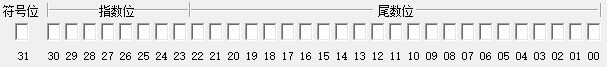那么一个单精度十进制浮点数转二进制浮点数的规则是如何的呢？假设这里有一个小数为3.625，那么该小数对应的整数部分就是11，小数部分就是101，那么该数表示成二进制就是11.101，由于我们需要表示成浮点二进制数，那么小数点要向左移动一位，那么变为1.1101，那么对应的浮点二进制整数部分就是127+1=128=0x80,小数部分为1101，由于该数是整数，所以符号位为0，将上述数字如图对号入座，其余空余的地方补1，可得转换后的数据是：0100 0000 0110 1000 0000 0000 0000 0000，对应的十六进制表示就是0x40680000，即3.625的单精度浮点二进制数表示就是0x40680000。

（2）双精度二进制浮点数存储格式如下图：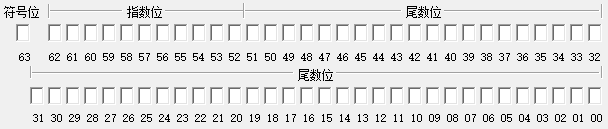那么一个双精度浮点数的转换规则是怎样的呢？其实和单精度浮点数的转换机制类似，由（1）3.625对应的二进制数为11.101，小数点左移一位后为1.1101，整数部分就为1023+1=1024=0x800，小数部分为1101，符号位为0，按如图格式对号入座，其余部分补0，得到转换后的的数为0100 0000 0000 1101 000 0000 0000 0000 0000 0000 0000 0000 0000 0000 0000 0000 0000=0x400D000000000000，即3.625的双精度浮点二进制数表示就是0x400D000000000000。

（3）便捷计算软件

网上可以找到浮点数转换的便捷计算软件，非常方便，如下图这个软件：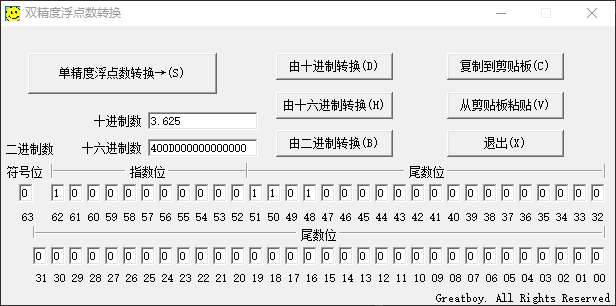展开全文Vivado IP核
• ## 计算机浮点数规格化表示

万次阅读 多人点赞 2017-12-27 17:48:37
说明在IEEE标准中，浮点数在内存中的表示是将特定长度的连续字节的所有二进制位按特定长度划分为符号域，指数域和尾数域三个连续域。 floatfloat类型在内存中占用的位数为: 1+8+23=32bits double1+11+52=64bits 第...浮点数 浮点数规格化
• 半精度二进制浮点数的大批量转换，发现没有现成的转换代码，就根据原理用matlab撸了一个，与在线转换器对比暂时没有发现bug，通过写blog的方式debug（小黄鸭调试法），也欢迎大家帮我找茬，一起debug. 半精度浮点数...机器学习 经验分享
• ## 浮点数运算规格化

千次阅读 多人点赞 2019-12-07 11:14:20
临近考试，计算机组成原理令人头疼不已，浮点数的运算规格化看书依旧是云里雾里，偶然看到一篇博客，写的很清晰明了，分享一下。 正文 在尾数用补码表示时，规格化浮点数应满足尾数最高数位与符号位不同，如...浮点数运算规格化
• 如何将十进制的浮点数 转换二进制浮点数,分为两部分： 1. 先将整数部分转换为二进制， 2. 将小数部分转换为二进制， 然后将整数部分与小数部分相加。 　以 20.5 转换为例，20转换后变为10100；0.5 要转换...
• 了解二进制浮点数的表示及其范围 如图所示表示的是一个阶码为N位，尾数为M位，且分别有符号位的二进制浮点数 首先要了解：阶码位为纯整数，通常为2^E (E为阶码表示为2^N) 所以阶码表示范围为:正负2^{2^[(N+1)-1]} ...经验分享 算法
• 二进制浮点数表示 sign 为符号位 exponent 为指数位（以2为底） fraction为分数部分 Value=sign*exponent*fraction 一、IEEE 754的前世今生 在IEEE 754出现前，各家计算机公司自己使用自己的数值表示标准，当时...
• 1.十进制转二进制方法  十进制数转换为二进制数 十进制数转换为二进制数时，由于整数和小数的转换方法不同，所以先将十进制数的整数部分和小数部分分别转换后，再加以合并。  1.1十进制整数转换为二进制整数 ...
• 在看这篇文章之前，请先看下二进制规格化数判断的文章。 链接如下： https://blog.csdn.net/m0_56032189/article/details/115323070?spm=1001.2014.3001.5501； 如果你看完了上述文章，那我们就可以把二进制规格数的...补码
• 将十进制数转换为IEEE 单精度二进制浮点数（C代码） 介绍： IEEE 单精度浮点数分为三个部分 sign(符号位）exponent（8位） fraction（尾数 23位） 总共32位 这里举一个例子：例如将7转成IEEE单精度二进制...c语言
• 计算机基础：20、二进制--浮点数的乘除法运算浮点数乘法公式浮点数的除法公式例子 浮点数乘法公式 浮点数的除法公式 例子 浮点数的乘除法运算也是分成阶码运算、尾数运算、尾数规格化、舍入、溢出判断这几个过程的...
• ## 浮点数的二进制表示(IEEE 754标准)

千次阅读 多人点赞 2019-02-28 11:59:14
浮点数是我们在程序里常用的数据类型，它在内存中到底是怎么样的形式存在，是我了解之前是觉得好神奇，以此记录，作为学习笔记。现代计算机中，一般都以IEEE ...根据这个标准，我们来尝试把一个十进制浮点数转换...浮点数的二进制
• IEEE-754标准（32位）十六进制转十进制浮点数 #include<fstream> #include <sstream> #include <iostream> #include<math.h> using namespace std; double BtoD(string x) { double ans; ...
• 十进制整数转二进制 输入整数以补码形式显示 ...十进制浮点数转IE754格式二进制 IE754格式二进制 S 符号位:     1 表示negative负数 ; 0表示 positive正数 Exponent（阶码）:     规格化阶码范围为0000 0001 (-c++ 进制转换 十进制转二进制
• 虽然不是专业的CS出生，想想接触CS相关的东西也这么久了，居然不知道浮点数在内存中的表示是怎样的，实在是惭愧啊！于是最近查阅了相关资料，终于弄清了浮点数在内存中的表示，现在对相关的东西做一个总结。 　以下...二进制
• 1.将（32位）16进制IEEE-754标准浮点数就是用十六进制表示浮点，称为单精度浮点数。 float类型在内存中占4个字节（32位），最高位用于表示符号;在剩下的31位中，从右向左了8位用于表示指数，其余用于表示尾数。（一...
• 1.前几天，我在读一本C语言教材，有一道例题： #include <stdio.h> 　void main(void){ 　int num=9;... /* pFloat表示num的内存地址，但是设为浮点数 */ 　printf("num的值为：%d\n",num); /* ...浮点数 二进制 浮点数的二进制表示
• ## 浮点数转换二进制

千次阅读 2018-11-14 18:12:53
浮点数在计算机中存储也是以二进制的形式，遵循IEEE二进制算数标准；格式为： float : 符号位（首位）、指数位（8位）、尾数（23位） double：符号位（首位）、指数位（11位）、尾数（52位）   一 格式 符号位...浮点数 二进制
• 一、十进制数转换为二进制数 十进制数转换为二进制数时，由于整数和小数的转换方法不同，所以先将十进制数的整数部分和小数部分分别转换后，再加以合并。 1. 十进制整数转换为二进制整数 十进制整数转换为二进制...java
• 计算机基础：19、二进制--浮点数的加减法运算浮点数加减法1.1、对阶1.2、尾数求和1.3、尾数规格化（左移）1.4、尾数规格化（右移）1.4.1、舍入1.5、溢出判断2、浮点数加减法总结 浮点数加减法 浮点数加减法公式如下...
• 不应该用==号来判断浮点数是否相等,因为存在一个精度的问题 首先说一下原,反,补,移码. 移码其实就等于补码,只是符号相反. 对于正数而言,原,反,补码都一样, 对负数而言,反码除符号位外,在原码的基础上按位取反,补码...IEEE754 进制转换 十进制转换二进制
• 可能很多人都遇到过浮点数精度丢失的问题，下面以JavaScript为例。 1 - 0.9 = 0.09999999999999998 纳尼，不应该是0.1么，怎么变成0.09999999999999998呢？这就要从ECMAScript标准讲起了。 ECMAScript 并不像...
• &...所谓原码就是二进制定点表示法，即最高位为符号位，“0”表示正，“1”表示负，其余位表示数值的大小。 &amp;nbsp;&amp;nbsp;&amp;nbsp;&amp;nbsp;反码表示法规定：正数的反码
• 本篇是二进制系列第三篇，如若你有兴趣，请持续关注，后期会持续更新。其他文章列表如下:一、 精度如果你有看过《有趣的二进制》这篇文章，你就会明白进制(不局限于二进制)中的小数是如何表示。因为每种进制都有其...
•经验分享 java 后端...